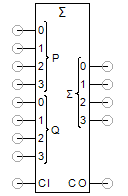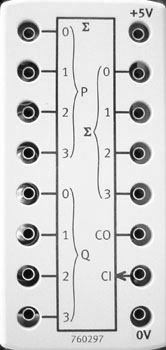This full adder can be used to add two 4-bit numbers. With the input CI (carry in), a previous carry can be included in the addition. The sum x is calculated from the inputs as follows:

x = CI + P0*2^0 + P1*2^1 + P2*2^2*P3*2^3 + Q0*2^0 + Q1*2^1 + Q2*2^2*Q3*2^3

The outputs S0, S1, S2, S3 and the output CO (carry out) represent the sum x bit-by-bit, which is composed as follows:

x = S0*2^0 + S1*2^1 + S2*2^2 + S3*2^3 + CO*2^4

The IC 74HC283 is used as a component in the training package TP 1012 from Festo Didactic.